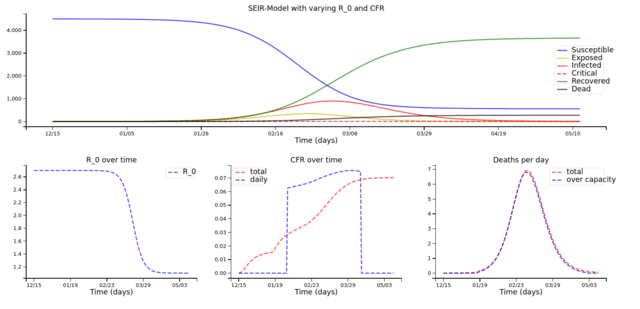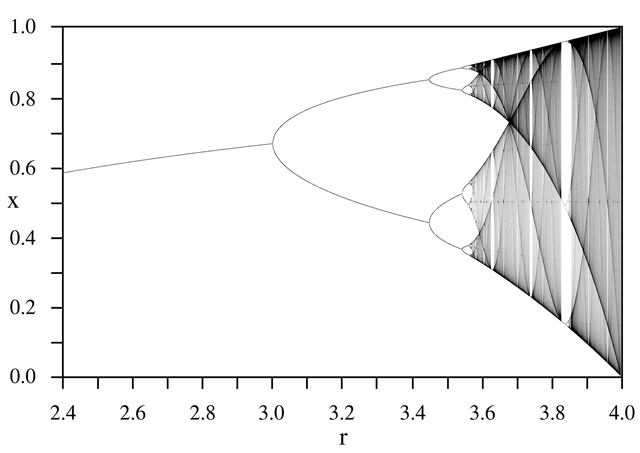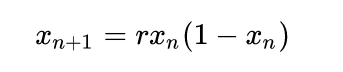# Covid-19 and chaos.

The first wave of Covid-19 was underestimated mainly because the Chinese data were similar to those received from the Kremlin in the first days of Chernobyl: “everything is fine”. They talked (and still do, by the way) about something that was little more than an influence. And again (to read their numbers) it seems that the lockdown of the scarce 3% of the population, which occurred two months late, has literally vaccinated more than a billion people.

But now we have more data on its propagation, and we can begin to move beyond the eighth grade charts found in the press. Or rather: we can start adding more complex graphics.

Traditionally, the trend of the time of an epidemic is represented by a model that is the son of the SIR. The model makes use of three exponentials to model the famous “to flatten” curve.This is the model used so far, and so far it goes all too well. However, it explains the general trend, but does not explain some peculiarities of the data "in the field". For example:As you can see, there is clearly an oscillating component in the average course of the infection. This oscillating component is clearly harmonic, of period one (although sometimes it has a period of two – the smaller peak between two large peaks), and in my opinion that should also be explained. But to explain it, another model is needed, which is equivalent to the SIR as regards the average trend, but also capable of explaining period two.

Welcome to chaos theory. Today we talk about the Logistics Map.The logistic map is the fractal of an equation which is completely equivalent to the SIR model, given the adequate constants.

It is an equation that is written like this, as a series:The part (1-x) represents nothing more than the component of the SIR that decreases (due to the dead or already infected), X is the percentage (expressed as an interval in real life from 0 to one) of infected population. The logistic equation is a demographic model, exactly like that of the SIR, which expresses the non-Malthusian growth of the population, that is the growth of a population in an environment with limited resources, which has a certain mortality rate. what is called r in the logistic equation, biologists call it R0 . (It is important to point this out).

How do you read the forked map above, and what is its relationship with the SIR? It is a map, that is of the projection of space on itself, and as such it should not be read as a time-series, that is as a trend over time.

Each point on the map represents the outcome of an epidemic. It means that all possible epidemics (USA, Germany, Italy, etc) are a point of that graph. And if we divide a country into parts, that I know the US into states, each outcome of the epidemic will still be a point on the same map).

Each point is an attractor, which in simple terms we could indicate as "the final effect of the epidemic", or "where the epidemic wants to end up". The epidemic under lockdown is also a point on this map, because we have decreased the population at risk but the epidemic is still there, etcetera: each subset of the epidemic is still a point on the map. This is why it is said that it is a fractal: each sub-part still contains the whole map.

Well.

Let's go back to the swings. We have said that these are period one oscillations, that is, the system oscillates between two values, one high and one low. Well.

In which area does the logistic map oscillate between two values ​​(no more and no less?). This is the area that sees r (the R0 of biologists) between the value of 3 and the value of 3.47 …

But we have said that the "natural" R0 of the coronavirus is 2.6, so far. Well, no. The vast majority of viruses have a natural R0 of 2.6, but as far as the period 1 fluctuations tell us, there is a sub-tribe of that virus which has an R0 between 3 and 3.4.

It is a LESS numerous tribe, indeed it would seem LITTLE numerous, since it represents only an oscillation (some would say a harmonic) of the largest curve.

But it has an R0 ranging between 3 and 3.4. Which, in an exponential, is MUCH more dangerous than a 2.6.

But in the last few weeks, a double period has appeared. A double period is the one where the curve oscillates between 4 points, with a small peak followed by a large peak. In the logistic curve, the four-point bifurcation appears for r = 3.8 and corresponds to a situation with four attractors, ie epidemics can stabilize in four different ways.

Thus, there is another very ferocious coronavirus sub-tribe, even less numerous but even more ferocious than the second .

Now, perhaps you will feel comforted by the fact that so far these are few tribes. But the growth of these phenomena is exponential. Being few in number at first means little.

What does this tell us about the "second wave"? He tells us that it will be even more difficult to flatten the curve, and that its growth will be explosive, MUCH more than the first wave.

These subtribes are less numerous, but they grow in number much faster, and we are talking about exponentials.

# I therefore expect, very soon, an explosive proliferation of these two "subtribes" of viruses.

The logistic curve obviously does not allow us to say whether these two tribes, in addition to being explosive in terms of propagation, are also equally lethal. We know in principle that viruses do not benefit from the death of the host, so we are hopeful that an evolution of the virus will also lead to a lower mortality.

I hope that's the way it is, because otherwise the situation is going to get bad pretty soon.

At the level of speculation, I suppose you have wondered what an epidemic that has an r = 4 does. Why are there so many bifurcations there, right?

Exact. For r = 4 the attractors cover the whole plane. A virus that has r = 4 would be unstoppable no matter how lockdown is done, as it would have – every possible ability to spread. This is a theoretical situation (unless some biologist does not know such viruses, I'm curious) but in fact we would be in strong poop, because it would not be possible to stop the epidemic, since the next day the number of infected people it could be… any. From 0% to 100% of the population, with the same probability.

So no, exceeding the value of 3.8 is very difficult (also because as you can see in the logistic equation there is a stretch of stability between two very divergent values ​​and much larger than the average value), but honestly, a virus that has a subtribe with R0 between 3 and 3.4 by now since May, and probably another with R0 at 3.8, is worrying enough .

Even if it were not very deadly, given its capacity for infection, even the "Italian" lockdown would have little hope of stopping it. (some evolutionists might say it's an adaptation to the lockdown itself, but I have no theoretical tools to prove it)

Consequently, I really think so: the "second wave" is certain, it will appear with the first cold (which here in Germany has already arrived, so in my opinion we are almost there) and it will be much faster in spreading than the first.

“Fast” in exponential terms, commonly called “explosion”.